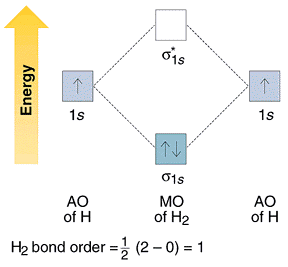# Molecular Orbitals of H2

Skills to Develop

Describe the hydrogen molecule in light of the following:

• $$\ce{H-H}$$
• $$\ce{H:H}$$
• Valence bond theory of $$\ce{H2}$$
• Molecular orbital theory of $$\ce{H2}$$
• Electron configuration of molecules

## Molecular Orbitals of H2

The hydrogen atom is the simplest atom, and its molecule $$\ce{H2}$$ is also the simplest molecule than monoatomic molecules such as $$\ce{He}$$ and $$\ce{Ne}$$, etc. Because of their simplicity, they have been extensively studied. Many theories have been developed using them as models. For example, this diagram from g(r,theta) Plots for a Diatomic Molecule in a Lennard-Jones Fluid by Aaron Lefohn shows one of the views of the $$\ce{H2}$$ molecule.Isn't it pretty!

### The Hydrogen Molecule

Atomic hydrogen gas is unstable, existing only at very high temperature. Diatomic hydrogen molecules, $$\ce{H2}$$, are formed at ordinary temperatures and pressures. Many models and theories have been proposed to explain the chemical bond, and here are some simplified forms of these theories, some of which may have been proposed as society began to speculate what is happening. A brief review is given below, and some of these items will be further explained.

• The hydrogen atom has a valency of 1 and it tends to form a chemical bond with another atom. It forms a covalent bond with another atom of the same kind to form $$\ce{H-H}$$.
• When people realized that the hydrogen atom has one electron, and that He has two electrons, they thought that an atom has a tendency to share electrons to achieve a closed shell as those of inert gases. Thus, its structure should be represented by $$\ce{H:H}$$, the Lewis dot structure.
• The invention of quantum mechanics to describe the electron configuration of atoms led to the electron configuration of 1s1 for hydrogen. This electron tends to find a partner, and sharing an electron with another hydrogen atom seems to make it more stable.
• Valence bond (VB) theory suggested 1s atomic orbitals of two $$\ce{H}$$ atoms overlap to form a chemical bond.
• Molecular orbital (MO) theory suggested atomic orbitals which not only overlap, but are simultaneously transformed into molecular orbitals with new energies and new electron distributions.

Some facts are known about hydrogen due to some careful experimental measurements. The internuclear distance is 74 pm, and the dissociation of an $$\ce{H-H}$$ bond into two atomic hydrogen atoms requires 7.2x10-19 J, (usually given as 435.9 kJ/mol).

### Molecular Orbitals of H2

The molecular orbital approach is one explanation for the $$\ce{H-H}$$ bond. This explanation is based on a mathematical model, hence it is a theory.

When the 1s wave functions of the two $$\ce{H}$$ atoms are linearly combined, we get a sigma (s) bonding orbital, denoted as s1s in the diagram here. This approach is called linear combination of atomic orbitals (LCAO), in the MO approach. In this approach, the sum of the two 1s orbitals (one for each atom) is the bonding orbital. In terms of wave mechanics, the two waves constructively interact. The difference of the two orbitals forms the antibonding orbital, s1s*, due to destructive interference. It is interesting to note that the anti bonding orbital is at a higher energy than the 1s atomic orbital. The energy level can be represented below:Each orbital accommodates two electrons, and the two electrons in $$\ce{H-H}$$ fill the s1s molecular orbital (MO). Obviously, as a result of the formation of the $$\ce{H2}$$ molecule, the energy of the system is lowered and becomes more stable.The quantum mechanics of the chemical bonding is a little complicated, but the logic and concept are as simple as described above. I have written a program for the DOS version of CAcT to plot electron densities of the s1s and s1s* electron densities, but the program cannot be executed on the web page. The execution of these types of simulations is sometimes shown during the lecture.

After the introduction of the molecular orbital theory for the hydrogen molecule, you might want to learn more about molecular orbital theory of diatomic molecules.

## Confidence Building Questions

1. What is the bond length (in pm) for the $$\ce{H-H}$$ molecule?

Hint: Length $$\ce{H-H}$$ bond is 74 pm, the shortest chemical bond.

Discussion: No need to memorize this value, but you have a general idea about bond length. Thus, the covalent radius for $$\ce{H}$$ is 37 pm.

2. Which one of the following statements is the best description of valence bond theory?
1. an overlap of atomic orbitals
2. satisfies the octet rule
3. count the number of VSEPR pairs
4. the overlap of atomic orbitals
5. atomic orbitals undergo a transformation

Hint: d.

Discussion: The simple version assumes that atomic orbitals do not change during the formation of chemical bonds. They merely overlap. Modern treatments of valence bond theory do accept the fact that atomic orbitals do change a little.

3. What is a molecular orbital of a molecule?

Hint: A molecular orbit is an energy state of the electron in the molecule.

Discussion: When atoms come together to form chemical bonds, new orbitals are formed. Molecular orbitals play the same role for molecules that atomic orbitals play for atoms.

4. Choose the best Lewis structure for $$\ce{HCN}$$:
1. $$\ce{H-C}\textrm{:::N:}$$
2. $$\ce{H-C}\textrm{::N::}$$
3. $$\ce{:H-C}\textrm{::N}:$$
4. $$\textrm{H::C::N:}$$

Hint: a.

Discussion: The triple bond between $$\ce{C}$$ and $$\ce{N}$$ is represented by three pairs of electrons.